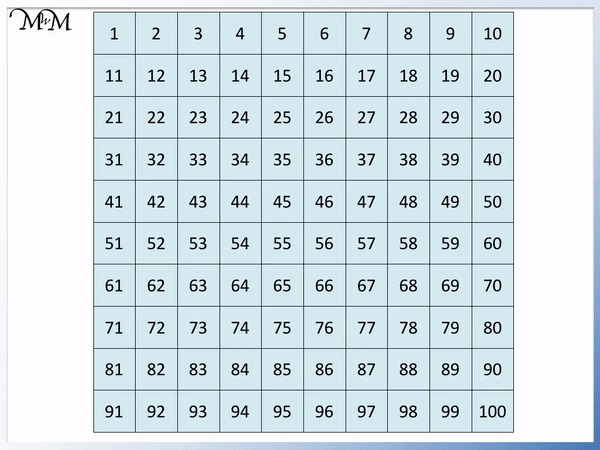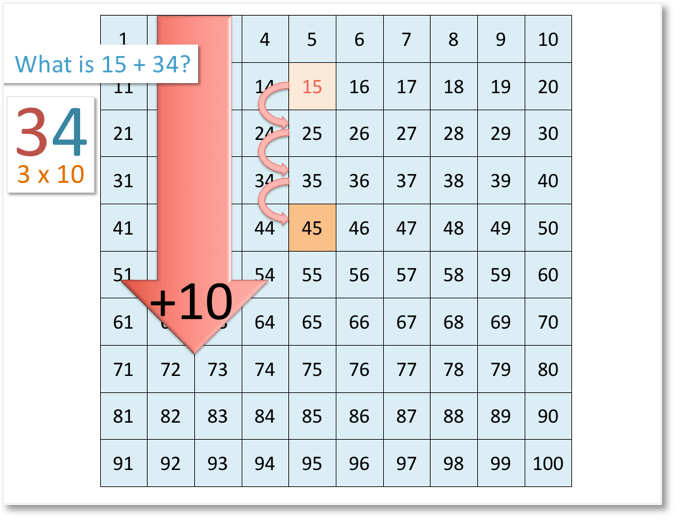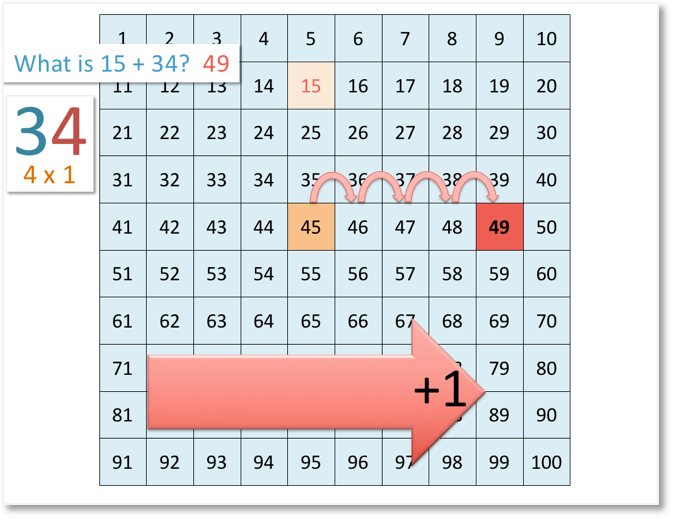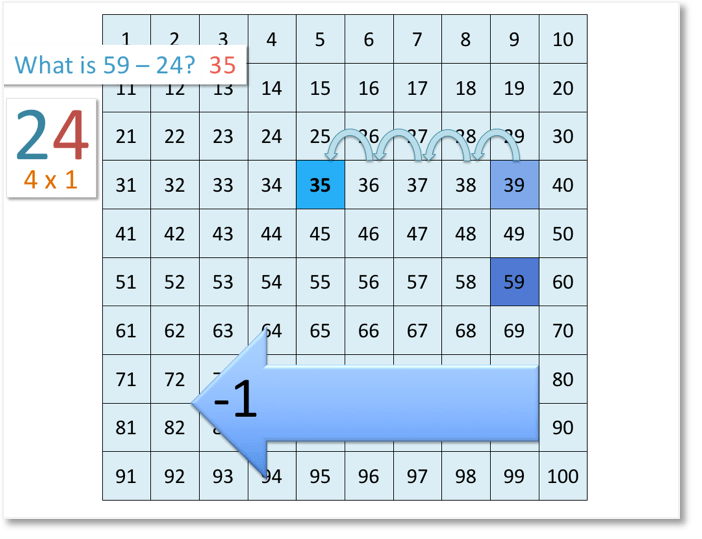# Adding 2-Digit Numbers on the Number Grid

Adding 2-Digit Numbers on the Number Grid• To add 10 on a number grid we move down one row.
• For example, 25 + 10 = 35, since we start at 25 and move down one row.
• To add 1 on the number grid we move right one column.
• For example, 56 + 1 = 57, since we start at 56 and move right one column.
To add 10 we move down one row.

To add 1 we move right one column.

#### Printable Number Grid 1 – 100• We are adding the 2-digit numbers 27 and 45.
• 45 is made up of 40 + 5.
• 40 is four lots of 10 and to add 40 we move down four rows.
• 27 + 40 = 67.
• We will now add the 5 by moving to the right.
• We add 3 and get to 70. To add one more, we move to the beginning of the next row.
• We arrive at 72 having added 5.
• 27 + 45 = 72.# How to Add 2-Digit Numbers using a Number Grid

To add 2-digit numbers using a number grid we can move down and right on our grid.

To add ten using the number grid, we move down on row of the grid.

To add one, using a number grid, we move one column to the right on the grid.

When we get to the end of a row and want to add one, we move to the beginning of a new row.

In this lesson, we will be looking at adding 2-digit numbers using the number grid for numbers between 1 and 100.

Here is our first example of adding 2-digit numbers:

What is 15 + 34?We’ll begin by looking at the tens column of 34.

We have 3 in the tens column. This means that we have 3 lots of 10.

So, we start at 15 and move 3 rows down the number grid.

We stop at 45.Next, we will add the units.

We have 4 in the units column of 34. This means that we have 4 lots of 1.

Every time we add one we move one column to the right.

So, we move 4 places to the right on the number grid.

We stop at 49.

Therefore,

15 + 34 = 49.

Here is another example of adding 2-digit numbers:

What is 27 + 45?We’ll begin by looking at the tens column of 45.

We have 4 in the tens column. This means that we have 4 lots of 10.

So, we start at 27 and move 4 rows down the number grid.

We stop at 67.Next, we’ll look at the units column of 45. We have 5 in the units column.

This means that we have 5 lots of 1. So, we want to move 5 places to the right on the number grid.

After moving 3 places, we reach the end of the row.

The next step is to move to the beginning of the next row.

We moved 3 places to get to the next row, now we need to move 2 more places to the right.

We stop at 72.

Therefore,

27 + 45 = 72.Now try our lesson on Subtracting 2-Digit Numbers on the Number Grid where we learn how to subtract 2-digit numbers using the hundred number grid.